#Compound Fractions: Problem 1Rule 27:
A compound fraction is sometimes called a mixed number.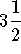,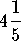, and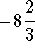are examples of compound fractions.Problem 1:
Convert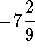to a simple fraction.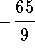Solution:
The compound fractioncan be written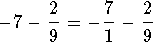.

Build the left fraction to an equivalent fraction with a denominator of 9.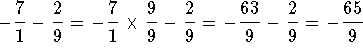.S.O.S MATHematics home page